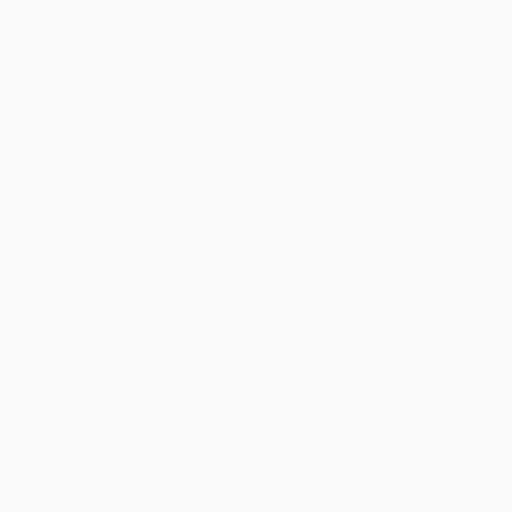CUET QUIZNumerical Ability

1 / 20

7589 - ? = 3434

2 / 20

Which of the following numbers is exactly divisible by 24?

3 / 20

How many terms are there in 2, 4, 8, 16, .............., 1024?

4 / 20

Which of the following is always odd?

5 / 20

If x and y are negative, then which of the following statement is/ are always true?

1. x + y is positive.

2. xy is positive.

3. x - y is positive.

6 / 20

5978 + 6134 + 7014 = ?

7 / 20

The digit in the units place of the product is 81 * 82 * ........ * 89 is :

8 / 20

9548 + 7314 = 8362 + ?

9 / 20

9572 - 4018 - 2164 = ?

10 / 20

9358 - 6014 + 312 = ?

11 / 20

39798 + 3798 + 378 = ?

12 / 20

1399 * 1399 = ?

13 / 20

The smallest number that must be added to 803642 in order to obtain a multiple of 11 is:

14 / 20

8756 * 99999 = ?

15 / 20

If a and b are both odd numbers, which of the following is an even number ?

16 / 20

Which one of the following is a prime number?

17 / 20

The value of P, when 4864 * 9P2 is divisible by 12, is:

18 / 20

360 * 17 = ?

19 / 20

The total number of even prime number is?

20 / 20

How many numbers between 400 and 600 begin with or end with a digit of 5?